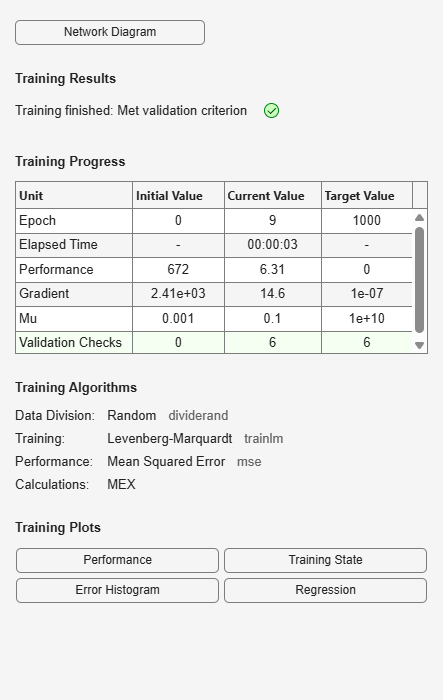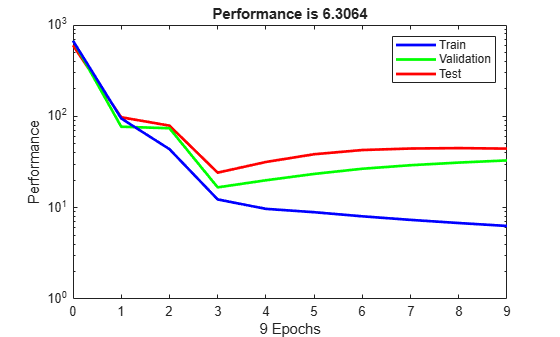## Analyze Shallow Neural Network Performance After Training

This topic presents part of a typical shallow neural network workflow. For more information and other steps, see Multilayer Shallow Neural Networks and Backpropagation Training. To learn about how to monitor deep learning training progress, see Monitor Deep Learning Training Progress.

When the training in Train and Apply Multilayer Shallow Neural Networks is complete, you can check the network performance and determine if any changes need to be made to the training process, the network architecture, or the data sets. First check the training record, `tr`, which was the second argument returned from the training function.

`tr`
```tr = struct with fields: trainFcn: 'trainlm' trainParam: [1x1 struct] performFcn: 'mse' performParam: [1x1 struct] derivFcn: 'defaultderiv' divideFcn: 'dividerand' divideMode: 'sample' divideParam: [1x1 struct] trainInd: [1x176 double] valInd: [1x38 double] testInd: [1x38 double] stop: 'Validation stop.' num_epochs: 9 trainMask: {[1x252 double]} valMask: {[1x252 double]} testMask: {[1x252 double]} best_epoch: 3 goal: 0 states: {1x8 cell} epoch: [0 1 2 3 4 5 6 7 8 9] time: [1x10 double] perf: [1x10 double] vperf: [1x10 double] tperf: [1x10 double] mu: [1x10 double] gradient: [1x10 double] val_fail: [0 0 0 0 1 2 3 4 5 6] best_perf: 12.3078 best_vperf: 16.6857 best_tperf: 24.1796 ```

This structure contains all of the information concerning the training of the network. For example, `tr.trainInd`, `tr.valInd` and `tr.testInd` contain the indices of the data points that were used in the training, validation and test sets, respectively. If you want to retrain the network using the same division of data, you can set `net.divideFcn` to `'divideInd'`, `net.divideParam.trainInd` to `tr.trainInd`, `net.divideParam.valInd` to `tr.valInd`, `net.divideParam.testInd` to `tr.testInd`.

The `tr` structure also keeps track of several variables during the course of training, such as the value of the performance function, the magnitude of the gradient, etc. You can use the training record to plot the performance progress by using the `plotperf` command:

`plotperf(tr)`The property `tr.best_epoch` indicates the iteration at which the validation performance reached a minimum. The training continued for 6 more iterations before the training stopped.

This figure does not indicate any major problems with the training. The validation and test curves are very similar. If the test curve had increased significantly before the validation curve increased, then it is possible that some overfitting might have occurred.

The next step in validating the network is to create a regression plot, which shows the relationship between the outputs of the network and the targets. If the training were perfect, the network outputs and the targets would be exactly equal, but the relationship is rarely perfect in practice. For the body fat example, we can create a regression plot with the following commands. The first command calculates the trained network response to all of the inputs in the data set. The following six commands extract the outputs and targets that belong to the training, validation and test subsets. The final command creates three regression plots for training, testing and validation.

```bodyfatOutputs = net(bodyfatInputs); trOut = bodyfatOutputs(tr.trainInd); vOut = bodyfatOutputs(tr.valInd); tsOut = bodyfatOutputs(tr.testInd); trTarg = bodyfatTargets(tr.trainInd); vTarg = bodyfatTargets(tr.valInd); tsTarg = bodyfatTargets(tr.testInd); plotregression(trTarg, trOut, 'Train', vTarg, vOut, 'Validation', tsTarg, tsOut, 'Testing')```The three plots represent the training, validation, and testing data. The dashed line in each plot represents the perfect result – outputs = targets. The solid line represents the best fit linear regression line between outputs and targets. The R value is an indication of the relationship between the outputs and targets. If R = 1, this indicates that there is an exact linear relationship between outputs and targets. If R is close to zero, then there is no linear relationship between outputs and targets.

For this example, the training data indicates a good fit. The validation and test results also show large R values. The scatter plot is helpful in showing that certain data points have poor fits. For example, there is a data point in the test set whose network output is close to 35, while the corresponding target value is about 12. The next step would be to investigate this data point to determine if it represents extrapolation (i.e., is it outside of the training data set). If so, then it should be included in the training set, and additional data should be collected to be used in the test set.

### Improving Results

If the network is not sufficiently accurate, you can try initializing the network and the training again. Each time your initialize a feedforward network, the network parameters are different and might produce different solutions.

```net = init(net); net = train(net, bodyfatInputs, bodyfatTargets); ```

As a second approach, you can increase the number of hidden neurons above 20. Larger numbers of neurons in the hidden layer give the network more flexibility because the network has more parameters it can optimize. (Increase the layer size gradually. If you make the hidden layer too large, you might cause the problem to be under-characterized and the network must optimize more parameters than there are data vectors to constrain these parameters.)

A third option is to try a different training function. Bayesian regularization training with `trainbr`, for example, can sometimes produce better generalization capability than using early stopping.

Finally, try using additional training data. Providing additional data for the network is more likely to produce a network that generalizes well to new data.